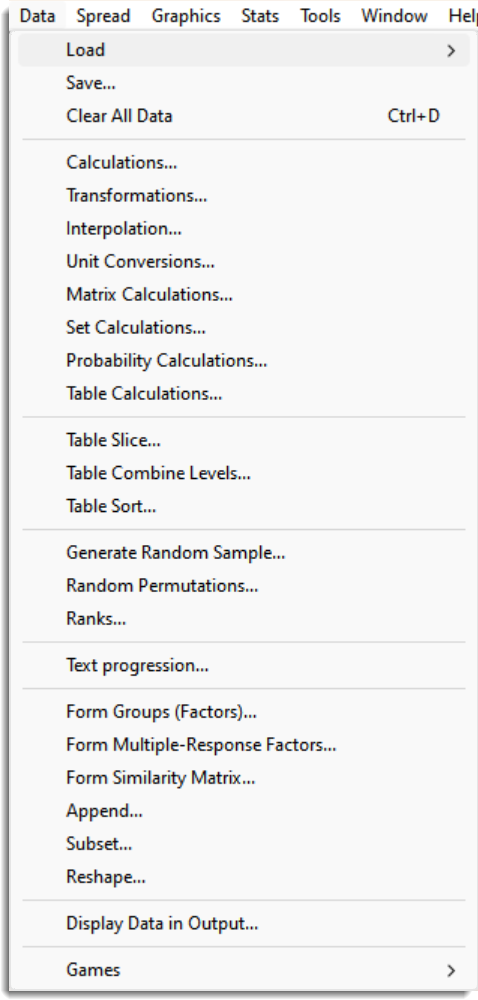This enables you to load data directly into Genstat from a variety of sources, as follows:

## Save

This lets you save the current Genstat data as a .gsv file, allowing you to reopen it later and continue where you left off. You can load data in this format back into Genstat using Data | Load | Resume. Note that the data are saved using a RECORD statement.

## Clear All Data

This deletes all current data structures from Genstat, by issuing an ENDJOB command. Data in open spreadsheet windows can be restored to Genstat using the Spread | Update menu.

## Calculations

Calculates new data using any general expression.

## Transformations

Transforms a data variate according to a transformation chosen from a list of common transformations.

## Interpolation

Interpolate to predict values at intermediate points in (X,Y) data.

## Unit Conversions

Performs standard unit conversions for different types of measurements.

## Matrix Calculations

Performs matrix calculations using a subset of functions from the general Calculations menu.

## Set Calculations

Performs Boolean arithmetic on sets contained within variates.

## Probability Calculations

Performs probability calculations for a range of discrete and continuous distributions.

## Table Calculations

Performs calculations with tables to create new tables.

## Table Slice

Takes a slice of a table to produce a new table with a subset of the levels of the original table.

## Table Combine Levels

Combines levels in a table to produce a new table with fewer levels.

## Table Sort

Sorts levels in a table to produce a reordered table.

## Generate Random Sample

Generates a random sample from a chosen distribution.

## Random Permutations

This performs a random permutation of the units for a set of factors and/or variates.

## Ranks

Calculates the ranks of values within a sample.

## Text progression

Form a text progression where the items in a text are created by a sequence of characters with a common offset (e.g. AA, AB, .. ZZ).

## Form Groups (Factors)

Converts data from a variate or text into a factor.

## Form Multiple-response factors

Produces multiple-response factors from a set of variates or text structures coding individual responses.

## Form Similarity Matrix

Forms a symmetric matrix containing similarities between each pair of
observations based on data contained in a set of variates.

## Append

Appends a set of vectors of the same type (factor, text, or variate) into a single vector of the same type.

## Subset

Forms a new vector containing a subset-menu of the values of an existing one.

## Reshape

Reshapes a data set with classifying factors for rows and columns,
into a reorganized data set with new identifying factors

## Display Data in Output

This opens the Display Data in Output menu that allows the output of selected structures to the Output window.